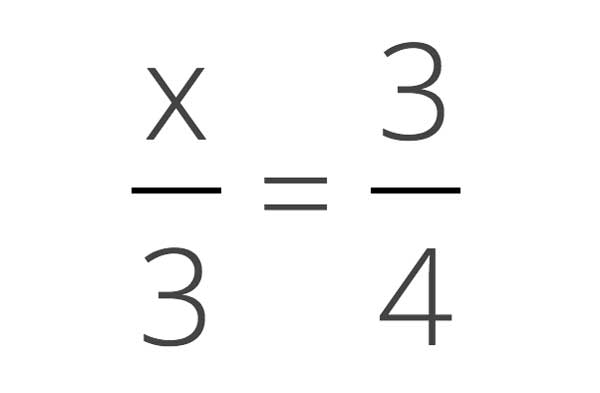# Solve For Unknown Fraction Calculator

Find an unknown numerator or denominator in a fraction using our simple calculator. Leave one field blank and the calculator will solve that field.

Enter 3 of the 4 fraction parts
Fraction One:
=
Fraction Two:

## Solution:

x=4.5

### Steps to Solve the Missing Value

Start by cross-multiplying the fractions
3x=23 = (3 × 3)=(x × 2)
Simplify the expression
3 × 3=2x
Multiply the known values
9=2x
Divide both sides by 2 to get x
92=2x2
92=x
4.5=x

## How to Solve Fractions in Algebraic Equations

You can solve for an unknown value x in algebraic equations containing fractions using a few simple steps.### Step One: Cross Multiply the Fraction

The first step to solving for the unknown numerator or denominator in a fraction is to cross-multiply the numerators and denominators. To cross-multiply, multiply each numerator by the denominator in the opposite fraction. This will create a new equation that is not a fraction and is easier to solve.

For example, cross-multiple the following equation to create a new equation without a fraction:
x3 = 34

x3 = 34
(4 × x) = (3 × 3)
4x = 9

### Step Two: Solve the Equation

The next step is to solve the resulting equation. To start, get x by itself by dividing both sides of the equation by the number in front of the x.

For example, let’s solve the equation 4x = 9.

4x = 9
4x4 = 94
x = 94

### Step Three: Reduce the Fraction

The final step is to reduce the fraction. Start by finding the greatest common factor of the numerator and the denominator. Then, divide both the numerator and denominator by the common factor. If you’re still not sure, check out our fraction simplifier to reduce your fraction.

If the numerator of the fraction is greater than 1 then you might want to turn the fraction into a mixed number. To do so, divide the numerator by the denominator. The quotient will be the whole number, the remainder will be the numerator, and the original denominator is the denominator.

For example, the fraction from the examples above cannot be simplified, but it can be turned into a mixed number.

94 = 9 ÷ 4
9 ÷ 4 = 2 R1
2 14